# Higher-Order Solution

Our zeroth-order solution is characterized by three undetermined flux-surface functions: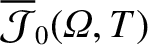,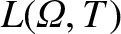, and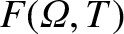. In order to determine the forms of these functions, we need to expand our model to higher order. (See Section 8.8.)

Expanding Equation (11.68) to third order in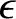, we obtain we obtain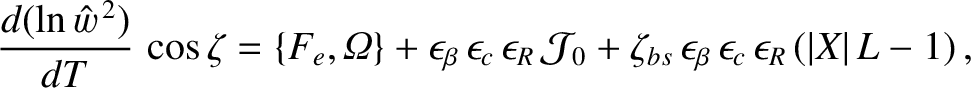(11.124)

where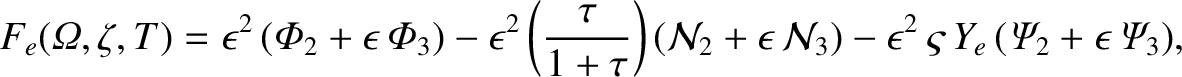(11.125)

and use has been made of Equations  (8.75), (11.95)–(11.99), (11.103), (11.104), (11.107), and (11.108). The flux-surface average of Equation (11.124) yields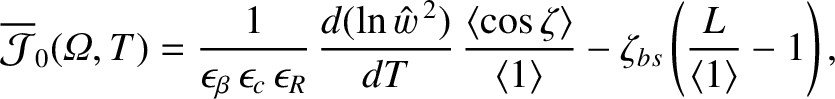(11.126)

where use has been made of Equations (8.68), (8.70), and (11.114), and (11.116). Note that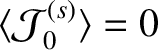, by symmetry. Hence,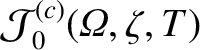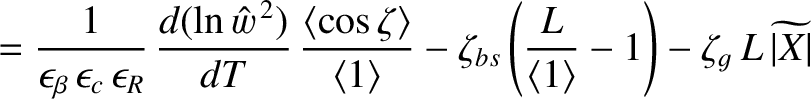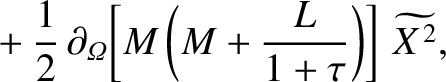(11.127)

where use has been made of Equation (11.116).

Expanding Equation (11.69) to first order in, we obtain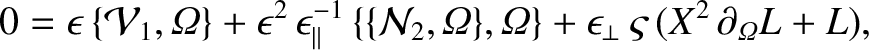(11.128)

where use has been made of Equations (11.95)–(11.99), (11.101), (11.103), and (11.104). The flux-surface average of the previous equation yields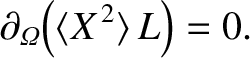(11.129)

We can solve the previous equation, subject to the boundary condition (11.105), to give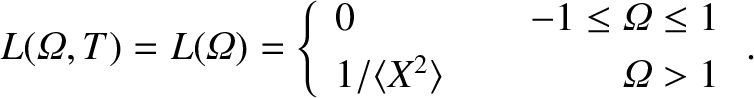(11.130)

Here, we have taken into account the previously mentioned fact that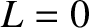within the island separatrix. Note that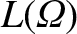is discontinuous across the island separatrix, which implies that the pressure gradient is also discontinuous across the separatrix. As discussed in Section 8.9, we expect this discontinuity to be resolved in a layer of characteristic thickness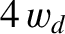on the separatrix [see Equation (8.84) and Table 8.1].

Expanding Equation (11.71) to second order in, we obtain we obtain

 0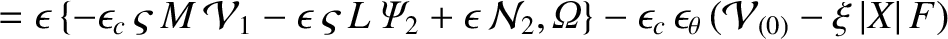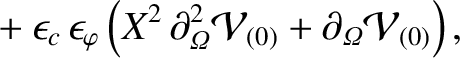(11.131)

where use has been made of Equations (11.95)–(11.99), (11.101), (11.103), (11.104), (11.107), (11.108), (11.111), and (11.113). The flux-surface average of the previous equation yields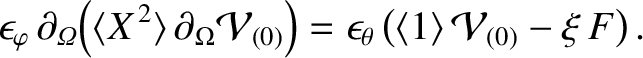(11.132)

According to Equation (11.121), recalling that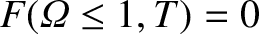, we get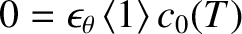(11.133)

inside the separatrix, which implies that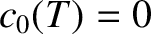. Hence, given that we have already concluded that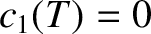, Equations (11.121) and (11.122) yield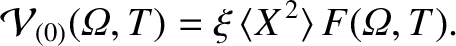(11.134)

The previous equation can be combined with Equation (11.132) to produce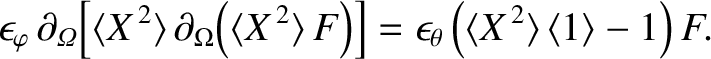(11.135)

Now, it is apparent from the previous equation that there is no discontinuity in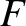across the separatrix. Thus, given that, it follows that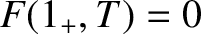. It is also clear from Equations (11.105), (11.109), and (11.113) that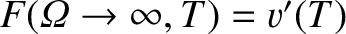. Thus, we can write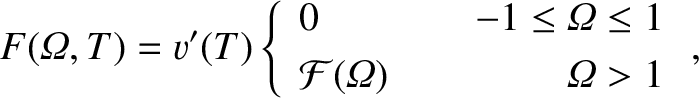(11.136)

where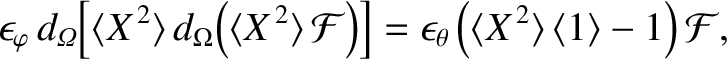(11.137)

and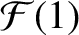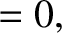(11.138)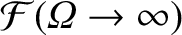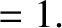(11.139)

Here,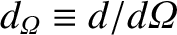.